# Contents

library(GWAS.BAYES)

# 1 Introduction

The GWAS.BAYES package provides statistical tools for the analysis of GWAS data. Currently the available functions allow analysis for selfing species such as rice and Arabidopsis Thaliana.

Our Bayesian pipeline for GWAS analysis selects significant SNPs in two stages. The first stage is the same as a typical statistical analysis of GWAS data: this stage fits as many linear mixed models as the number of SNPs, with each model containing one SNP as well as model components to account for correlation amongst the SNPs. These model fits yield a p-value for each SNP, and then multiple comparison correction such as the Bonferroni correction or the Benjamini and Hochberg FDR correction is used to obtain a set of significant SNPs. A typical GWAS analysis would then report this set of significant SNPs.

GWAS.BAYES goes one step further. The second stage of GWAS.BAYES performs Bayesian model search where the models are composed of the set of SNPs from the screening phase. This second stage allows the SNPs to compete with each other, and typically results in a much smaller set of SNPs. This second stage provides more information on which are the most promising SNPs for follow-up lab experiments.

To this extent GWAS.BAYES provides functions to:

• Preprocess SNP data and phenotype responses.
• Pre-screen individual SNPs in a traditional GWAS manner.
• Search for the Bayesian highest probability model including multiple significant SNPs.

This vignette explores three different examples to show how the functions provided in GWAS.BAYES implement the above points. The three case studies of interest are:

• A simulated data set with a population structure measured with principal components.
• A simulated data set with a kinship component using a centered IBS matrix.
• A real data set from A. Thaliana with a kinship component using a centered IBS matrix.

Each data set has a set of SNPs as well as a phenotype.

# 2 Functions

The functions implemented in GWAS.BAYES are described below:

• standardize Takes a matrix or dataframe where each column is a SNP with levels A, C, T, or G, and each row is a species/ecotypes/taxa. This returns a matrix of similar design but now the values of each column are 0’s or 1’s.
• aggregate_SNPs Aggregates the SNPs according to replications of the species/ecotypes/taxa.
• level_function Removes SNPs with Minor Allele Frequency less than a specified value.
• preprocess_SNPs Does what the standardize, aggregate_SNPs, and level_function do but wrapped all into one function.
• pca_function Computes principal components for controlling population structure/relatedness in the GWAS analysis.
• preselection Performs GWAS analysis and provides multiple options for controlling population structure/relatedness as well as different corrections for p-values.
• resids_diag Takes the significant SNPs from the GWAS analysis and assesses the distribution of the residuals. Users should use this function to make sure the assumptions of the GWAS analysis are met.
• postGWAS Searches for the Bayesian highest probability models that include multiple SNPs using a genetic search algorithm.
• cor_plot Creates a plot that examines correlations between specific sets of SNPs.

# 3 Model/Model Assumptions

To fully understand the package GWAS.BAYES some brief model details are required. The model for GWAS studies used in this package is

$\begin{equation*} \textbf{y} = X \boldsymbol{\beta} + Z \textbf{u} + A \textbf{q} + \boldsymbol{\epsilon} \ \text{where} \ \boldsymbol{\epsilon} \sim N(\textbf{0},\sigma^2 I) \ \text{and} \ \textbf{q} \sim N(\textbf{0},\sigma^2 \tau K) \end{equation*}$

where

• $$\textbf{y}$$ is the phenotype response.
• $$X$$ is the matrix of SNPs (single nucleotide polymorphisms).
• $$\boldsymbol{\beta}$$ is the vector of regression coefficients that contains the effects of the SNPs.
• $$Z$$ is a matrix with the population structure (eg. principal components).
• $$\textbf{u}$$ is a vector of coefficients related to the population structure.
• $$A$$ is an incidence matrix projecting the random effects associated with the kinship structure.
• $$\textbf{q}$$ is a vector of random effects associated with the kinship structure.
• $$\boldsymbol{\epsilon}$$ is the error vector.
• $$\sigma^2$$ is the variance of the errors.
• $$\tau$$ is a parameter related to the variance of the random effects.
• $$K$$ is the kinship matrix.

Not all GWAS models have to be comprised of a kinship matrix (relatedness) or principal components (population structure) but in some instances these structures are required to provide accurate results. A common issue with including the kinship component is the increased computational cost of this addition. GWAS.BAYES utilizes Efficient Mixed-Model Association (EMMA) techniques (Kang et al. 2008) to speed up computation. To get accurate results the assumptions of the model must be met as well. The model listed above assumes that $$\pmb{\epsilon}$$ follows a normal distribution. The vignette highlights how to assess this assumption.

# 4 Two Simulated Data sets

This section explores simulated data mimicking the selfing species A. Thaliana. We explore two simulated datasets where one data set has no kinship structure while the other data set does. In both simulated datasets the first five SNPs are the only SNPs that influence the response i.e. the first five SNPs are the only non-null SNPs. The datasets were created assuming that all SNPs are independent of each other.

## 4.1 Data with Population structure measured with principal components

### 4.1.1 Preprocessing Data

First we will discuss the format of the raw data as well as how to transform the data using functions available in the the GWAS.BAYES package.

data("vignette_lm_dat")
#>   Phenotype SNP1 SNP2 SNP3 SNP4 SNP5 SNP6 SNP7 SNP8 SNP9
#> 1  2.849069    G    T    A    A    T    C    G    C    G
#> 2  2.931406    G    T    A    A    T    C    G    C    G
#> 3  2.375999    G    T    A    A    T    C    G    C    G
#> 4  3.573713    G    T    A    A    T    C    G    C    G
#> 5  2.631515    G    T    A    A    T    C    G    C    G
#> 6  3.322648    G    T    A    A    T    C    G    C    G
Y <- vignette_lm_dat$Phenotype SNPs <- vignette_lm_dat[,-1] The SNPs can either be imported as strings or factors. To prepare SNPs for the analysis the data must be taken from its raw state (above) into a finer state to speed up analysis. First, apply the standardize function to transform the SNPs to {0,1}. This can be done in two ways. The first way is alphabetically; meaning that if the letter comes first in the alphabet then that would be a 1 while the letter that comes second in the alphabet would be a 0. The second way to do this is by major and minor allele, the major allele becomes a 1 and the minor allele becomes a 0. Note either method will lead to the same significant SNPs; the two will differ by the interpretation of the results. The default method is method = "major-minor" and the possible methods are method=c("major-minor","alphabetical"). SNPs <- standardize(SNPs = SNPs,method = "major-minor",number_cores = 1) SNPs[1:6,1:10] #> [,1] [,2] [,3] [,4] [,5] [,6] [,7] [,8] [,9] [,10] #> [1,] 1 0 1 1 1 0 1 1 1 1 #> [2,] 1 0 1 1 1 0 1 1 1 1 #> [3,] 1 0 1 1 1 0 1 1 1 1 #> [4,] 1 0 1 1 1 0 1 1 1 1 #> [5,] 1 0 1 1 1 0 1 1 1 1 #> [6,] 1 0 1 1 1 0 1 1 1 1 As in most GWAS studies, we aggregate both the SNPs and the phenotype over the replications of each species/ecotypes/taxa to speed up computation. The aggregate_SNPs function takes both the SNP matrix and the phenotype response and returns a list with the aggregated SNP design and the aggregated phenotypes. aggregate_list <- aggregate_SNPs(SNPs = SNPs, Y = Y) SNPs <- aggregate_list$SNPs
Y <- aggregate_list$Y The next step is to clean up the standardized SNP data in preparation for the preselection function. If this step is not taken the preselection function would break because it cannot give a p-value for a SNP that has the same allele for all species/ecotypes/taxa in the dataset. The level_function function will clean up the standardized SNPs by removing all SNPs with minor allele frequency less than or equal to the input value MAF. To keep all SNPs with minor allele frequency larger than zero, set MAF = 0. The function will return a list where the first element is a standardized SNP matrix and the second element is a vector of TRUE and FALSE that will be TRUE if the corresponding SNP is kept and FALSE if the SNP is removed. The default value for MAF is MAF = 0.01. level_list <- level_function(SNPs = SNPs, MAF = 0.01) SNPs <- level_list$SNPs
level_list$SNPs_Dropped #>  "None" dim(SNPs) #>  270 1000 Looking at the output above we can see that no SNPs had minor allele frequency less than 0.01 so the new matrix returned by the level function has the original 1000 SNPs. A user can compute all the above preprocessing steps using the preprocess_SNPs function. An example is highlighted below. fullPreprocess <- preprocess_SNPs(SNPs = vignette_lm_dat[,-1], Y = vignette_lm_dat$Phenotype,MAF = 0.01,number_cores = 1,
na.rm = FALSE)
all.equal(fullPreprocess$SNPs,SNPs) #>  TRUE all.equal(fullPreprocess$Y,Y)
#>  TRUE
fullPreprocess$SNPs_Dropped;level_list$SNPs_Dropped
#>  "None"
#>  "None"

The results above highlight how both methods of data preparation produce identical results.

Next, we estimate population structure through the computation of principal components. This is a common tool used in GWAS studies to eliminate the number of false positives. The pca_function function takes three inputs the matrix of standardized SNPs as created above, the number of principal components you would like in your model, and if you would like to plot the percent variation explained by the principal components. Make sure to utilize the plot_it variable within the function to fully understand how many principal components are needed to control for false positives. The function will return a matrix that has the same number of rows as the matrix you inputted and number of columns equal to the number of principal components.

principal_comp <- pca_function(SNPs = SNPs,number_components = 3,
plot_it = TRUE)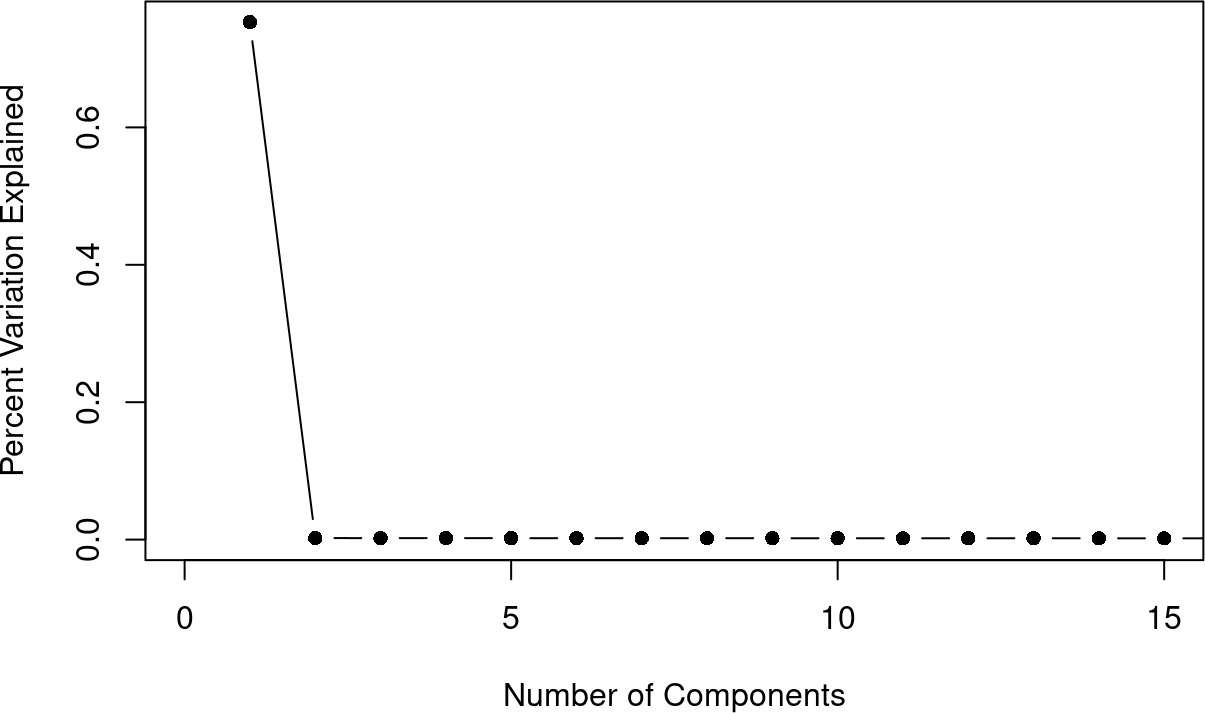Figure 1: Percent Variation in the Response explained by the principal components

Figure 1 indicates that one principal component is enough to capture most of the variation in the SNP data. Thus, we save the first principal component for later analysis using the below command.

principal_comp <- as.matrix(principal_comp[,1])

### 4.1.2 Pre-screen Step

The preselection function is based on a more typical GWAS analysis. This will look at each SNP individually with associated principal components or the potential kinship structure. One can find significant SNPs using p-values or BICs (frequentist = TRUE for p-values and frequentist = FALSE for BICs); from this, different multiple comparison corrections can be used for the p-values (controlrate). The BICs only use the Bayesian false discovery correction.

#### 4.1.2.1 Bonferroni Correction

Using the type 1 error rate .05.

Significant_SNPs_Bonf <- preselection(Y = Y, SNPs = SNPs,number_cores = 1,
principal_components = principal_comp,frequentist = TRUE,
controlrate = "bonferroni",threshold = .05,kinship = FALSE,
info = FALSE) #Bonferroni Correction
sum(Significant_SNPs_Bonf$Significant) #Five Significant SNPs #>  5 which(Significant_SNPs_Bonf$Significant == 1)
#>  1 2 3 4 5

The pre-screen step finds that the first 5 SNPs are significant, which are the true set of SNPs for the simulated data set.

We can assess the residuals to ensure that no transformation of the phenotype response is needed. To do this call the resids_diag function; this function will compute the model with the significant SNPs from the preselection step and will return a plot of the residuals and the results of a Shapiro-Wilk test for normality.

resids_diag(Y = Y,SNPs = SNPs,
significant = Significant_SNPs_Bonf$Significant, kinship = FALSE,principal_components = principal_comp, plot_it = TRUE)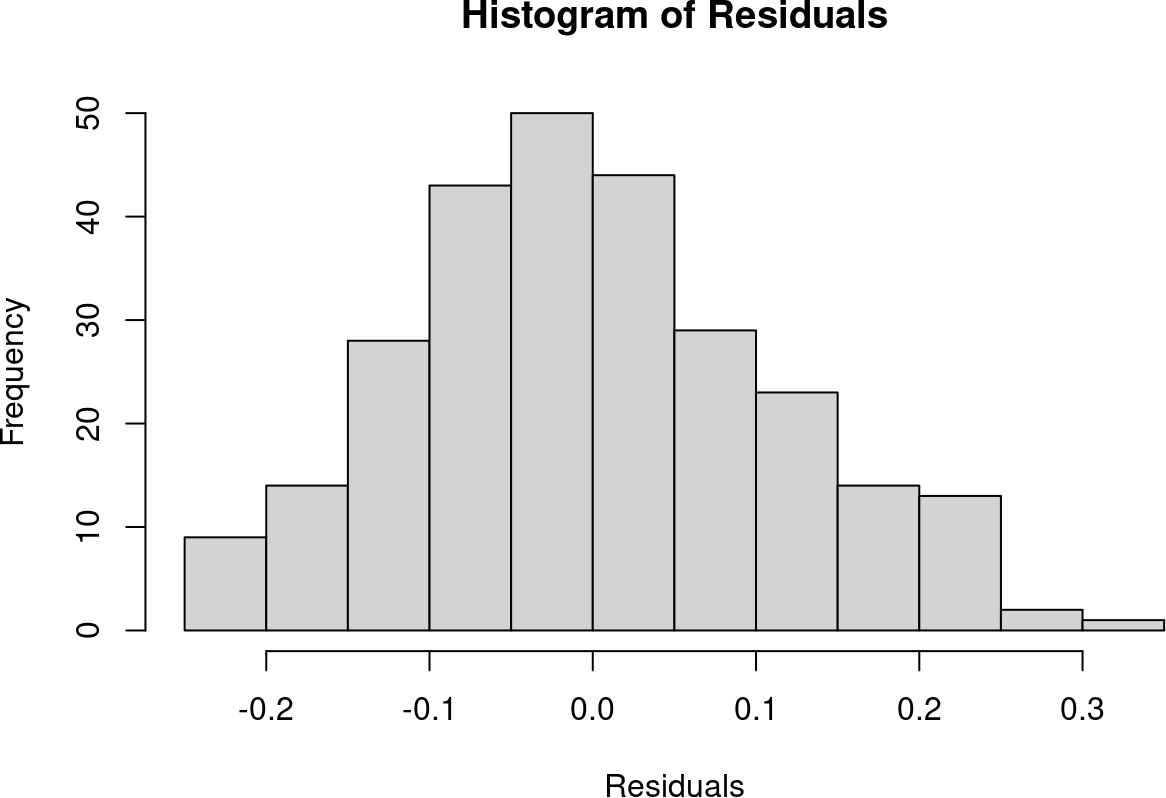#> #> Shapiro-Wilk normality test #> #> data: resids #> W = 0.9918, p-value = 0.1391 The Shapiro-Wilk test has a p-value of 0.1391. Therefore, there is no evidence that the residuals are not normally distributed. This suggests the Gaussian assumption is met and no transformation of the phenotype is warranted. The cor_plot function creates a plot to examine the correlations between significant SNPs. cor_plot(SNPs = SNPs, significant = Significant_SNPs_Bonf$Significant,
info = FALSE)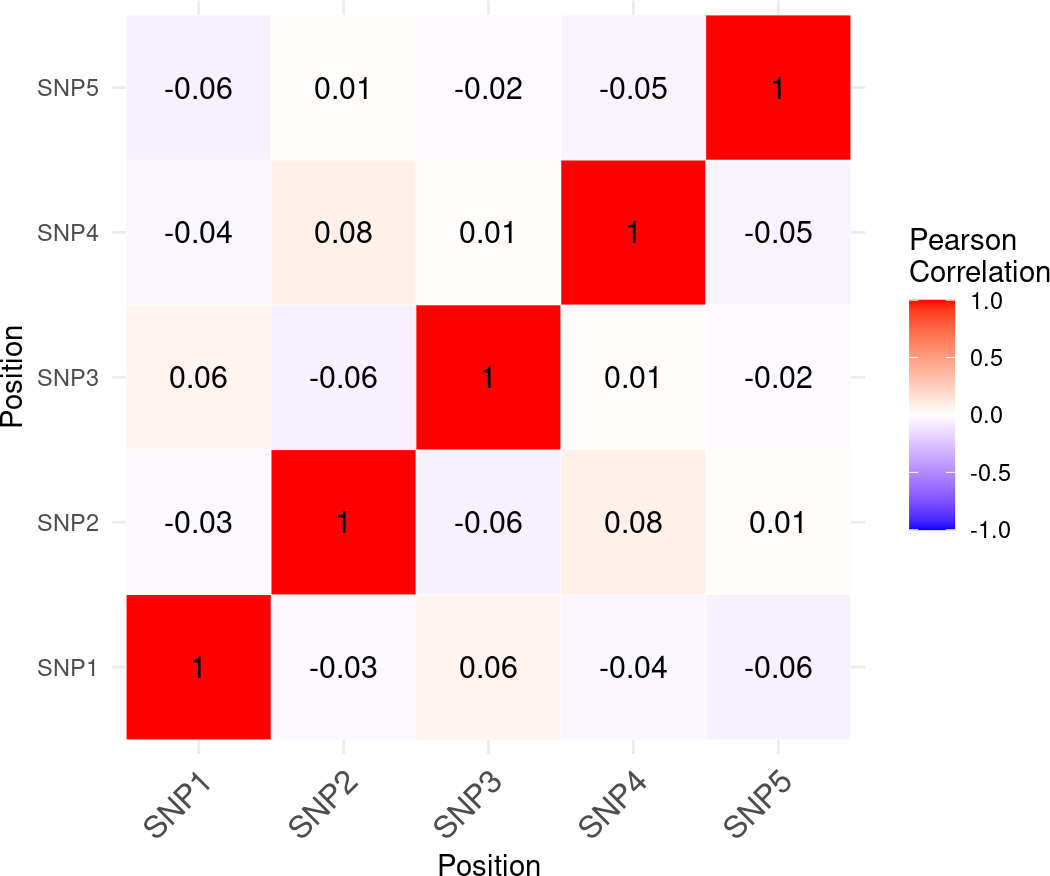This plot highlights the correlations between pairs of SNPs with the diagonal showing correlation between the same SNP.

#### 4.1.2.2 False Discovery Correction

The GWAS.BAYES package includes other methods of controlling multiple comparisons including the false discovery method proposed by Benjamini and Hochberg (1995). To see a full list of methods the package uses for frequentist multiple comparison corrections, type ?p.adjust and explore the p.adjust.methods section. In the example below the Benjamini-Hochberg correction is used (controlrate = "BH") with a 0.05 type 1 error rate.

Significant_SNPs_FDR <- preselection(Y = Y, SNPs = SNPs,number_cores = 1,
principal_components = principal_comp,frequentist = TRUE,
controlrate = "BH",threshold = .05,kinship = FALSE,info = FALSE)
sum(Significant_SNPs_FDR$Significant) #Five Significant SNPs #>  5 which(Significant_SNPs_FDR$Significant == 1)
#>  1 2 3 4 5

The pre-screen step with the Benjamini-Hochberg FDR correction also correctly finds that the first 5 SNPs are significant.

#### 4.1.2.3 Bayesian False Discovery Correction

GWAS.BAYES can also use Bayesian multiple comparison corrections such as the Bayesian False Discovery Correction (Newton et al. 2004). The example below uses equal weight on the null and alternative hypotheses (nullprob and alterprob respectively).

Significant_SNPs_BFDR <- preselection(Y = Y, SNPs = SNPs,number_cores = 1,
principal_components = principal_comp,frequentist = FALSE,nullprob = .5,
alterprob = .5,threshold = .05,kinship = FALSE,info = FALSE)
sum(Significant_SNPs_BFDR$Significant) #Five Significant SNPs #>  5 which(Significant_SNPs_BFDR$Significant == 1)
#>  1 2 3 4 5

The Bayesian False Discovery Correction also correctly finds that the first five SNPs are significant.

### 4.1.3 Bayesian Model Selection

The function postGWAS implements the second stage of GWAS.BAYES, which performs Bayesian model search where the set of candidate SNPs obtained from the screening phase compete with each other. The resulting best models typically contain a much smaller set of SNPs, which are the most promising SNPs for follow-up lab experiments.

The function below uses the results from the pre-screen step with the Bonferroni Correction. Note this is implemented with the significant argument.

GA_results <- postGWAS(Y = Y,SNPs = SNPs,number_cores = 1,
significant = Significant_SNPs_Bonf$Significant, principal_components = principal_comp,maxiterations = 100, runs_til_stop = 10,kinship = FALSE,info = FALSE) GA_results #>$Models
#>  "Model 1: y ~ SNP1 + SNP2 + SNP3 + SNP4 + SNP5 Posterior Prob. of 1"
#>
#> $Solution #> SNP1 SNP2 SNP3 SNP4 SNP5 #> [1,] 1 1 1 1 1 The exhaustive search suggests that the best model is the model with the first 5 SNPs again matching the correct settings for this simulated data set. In addition, the posterior probability of this model is 1, which provides further confirmation that these 5 SNPs are the most promising for follow up lab experiments. ## 4.2 Data with Population structure measured with a kinship matrix Another feature in the GWAS.BAYES package is the ability to model kinship. The kinship matrix defines relatedness between individuals or species in the analysis. Inclusion of random effects with kinship covariance matrix is a very popular tool to aid in the reduction of false positives in GWAS analyses. We will show how to calculate kinship using the function A.mat from the rrBLUP package (Endelman 2011) and use our package with the kinship structure. The function A.mat takes as an argument the matrix of standardized SNPs similar to the pca_function above. Note that since most GWAS analyses for selfing species have replications of the same ecotype, therefore having the same exact SNP structure, the A.mat function computes a kinship matrix between all different ecotypes with different SNP structure. data("vignette_kinship_dat") head(vignette_kinship_dat[,1:10]) #> Phenotype SNP1 SNP2 SNP3 SNP4 SNP5 SNP6 SNP7 SNP8 SNP9 #> 1 0.9370809 G C T C A T T A G #> 2 1.0153545 G C T C A T T A G #> 3 0.6490744 G C T C A T T A G #> 4 0.6758369 G C T C A T T A G #> 5 0.8911326 G C T C A T T A G #> 6 0.9906855 G C T C A T T A G ### 4.2.1 Preprocessing Data Following the steps that were laid out above: Y <- vignette_kinship_dat$Phenotype
SNPs <- vignette_kinship_dat[,-1]
fullPreprocess <- preprocess_SNPs(SNPs = SNPs, Y = Y, MAF = 0.01,
number_cores = 1,na.rm = FALSE)
SNPs <- fullPreprocess$SNPs Y <- fullPreprocess$Y
fullPreprocess$SNPs_Dropped #>  "None" Calculate the kinship matrix (k) with the rrBLUP package. We can parallelize this using the n.core argument in A.mat. As mentioned above, the rrBLUP package uses the centered IBS method. library(rrBLUP,quietly = TRUE) k <- A.mat(SNPs,n.core = 1) dim(k) #>  270 270 ### 4.2.2 Pre-screen Step To implement the model with a kinship structure the variable kinship must be set to kinship = k where k is the kinship matrix. #### 4.2.2.1 Bonferroni Correction Using a .05 type 1 error rate. Significant_SNPs_Bonf <- preselection(Y = Y, SNPs = SNPs,number_cores = 1, principal_components = FALSE,frequentist = TRUE,controlrate = "bonferroni", threshold = .05,kinship = k, info = FALSE) sum(Significant_SNPs_Bonf$Significant)#Four Significant SNPs
#>  5
which(Significant_SNPs_Bonf$Significant == 1) #>  1 2 3 4 5 The first 5 SNPs are significant using the pre-screen step matching the correct setting (first five SNPs were set to be non-null). We can easily assess the residuals when there is a kinship structure. Simply set the kinship value equal to the kinship matrix (kinship = k). resids_diag(Y = Y,SNPs = SNPs,significant = Significant_SNPs_Bonf$Significant,
kinship = k,principal_components = FALSE,plot_it = TRUE)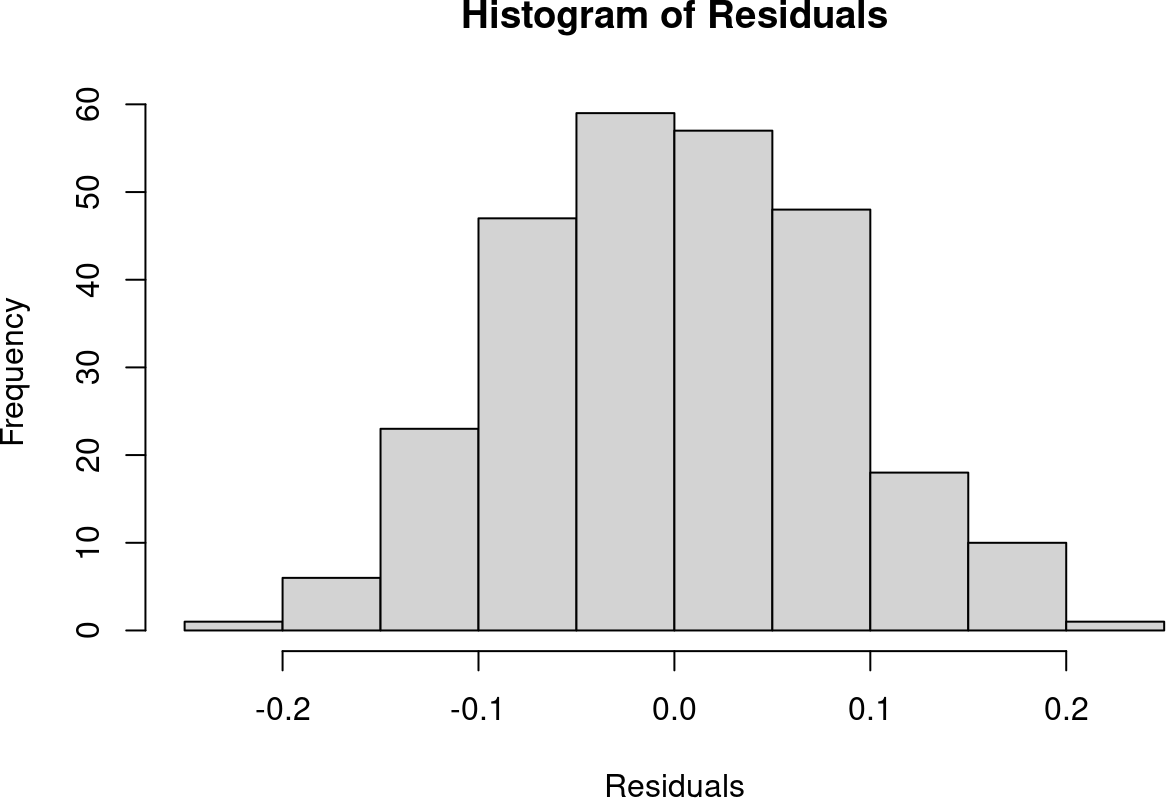#>
#>  Shapiro-Wilk normality test
#>
#> data:  resids
#> W = 0.99422, p-value = 0.3935

The other methods of controlling multiple comparisons are described below to give the reader an idea of the differences in results.

#### 4.2.2.2 False Discovery Correction

Using the type 1 error rate of .05.

Significant_SNPs_FDR <- preselection(Y = Y, SNPs = SNPs,number_cores = 1,
principal_components = FALSE,frequentist = TRUE,controlrate = "BH",
threshold = .05,kinship = k, info = FALSE)
sum(Significant_SNPs_FDR$Significant) #Six Significant SNPs #>  5 which(Significant_SNPs_FDR$Significant == 1)
#>  1 2 3 4 5

The pre-screen step for the Benjamini-Hochberg FDR Correction indicates that SNPs 1, 2, 3, 4, and 5 are significant. This identified the first 5 SNPs correctly.

#### 4.2.2.3 Bayesian False Discovery Correction

Using equal weight on the null and alternative hypotheses.

Significant_SNPs_BFDR <- preselection(Y = Y, SNPs = SNPs,number_cores = 1,
principal_components = FALSE,frequentist = FALSE,nullprob = .5,
alterprob = .5,threshold = .05,kinship = k, info = FALSE)
sum(Significant_SNPs_BFDR$Significant) #4 Significant SNPs #>  5 which(Significant_SNPs_BFDR$Significant == 1)
#>  1 2 3 4 5

Like the Bonferroni correction, the pre-screen step for the Bayesian False Discovery Correction suggests the first 5 SNPs are significant.

### 4.2.3 Bayesian Model Selection

We now perform Bayesian model selection using as candidate SNPs the significant SNPs decided by the Benjamini-Hochberg FDR. To include the kinship structure in the model, we again use the argument kinship = k.

GA_results <- postGWAS(Y = Y,SNPs = SNPs,number_cores = 1,
significant = Significant_SNPs_FDR$Significant, principal_components = FALSE,kinship = k, info = FALSE) GA_results #>$Models
#>  "Model 1: y ~ SNP1 + SNP2 + SNP3 + SNP4 + SNP5 Posterior Prob. of 1"
#>
#> $Solution #> SNP1 SNP2 SNP3 SNP4 SNP5 #> [1,] 1 1 1 1 1 The genetic search correctly proposes the model with the first 5 SNPs as the best model. The posterior probability for this model was the highest out of all models looked at. This can be interpreted as out of the models looked at Model 1 was the best model. # 5 A. Thaliana Analysis We highlight a real data analysis of A. Thaliana from the paper “Genetic Components of Root Architecture Remodeling in Response to Salt Stress” published in the journal Plant Cell (Julkowska et al. 2017). The article studies phenotype responses of A. Thaliana to salt stress. The phenotype studied in this vignette is the ratio of average lateral and main root length at low salt stress conditions (75 mM NaCl). This GWAS analysis is highlighted in figure 3 in their paper. The paper identifies a region of significant SNPs between chromosome 4 and chromosome 5 using a model that controls for relatedness using a kinship matrix. For illustration, the data set included in this vignette has the last 500 SNPs of chromosome 4, the first 500 SNPs of chromosome 5, as well as 500 additional SNPs randomly selected. ## 5.1 Preprocessing Data First we use the preprocess_SNPs function to preprocess the data: data("RealDataSNPs_Y") Y <- RealDataSNPs_Y$Phenotype
SNPs <- subset(RealDataSNPs_Y,select = -c(Phenotype))

fullPreprocess <- preprocess_SNPs(SNPs = SNPs,Y = Y,
MAF = 0.01,number_cores = 1,na.rm = FALSE)
SNPs <- fullPreprocess$SNPs Y <- fullPreprocess$Y
fullPreprocess$SNPs_Dropped #>  231 924 981 1372 1481 Two things are worth notice. First, the data set used in this example has no replications, so if one were using the individual functions standardize, aggregate_SNPs, and level_function, the aggregate_SNPs function would not be needed. Second, note that the SNPs_Dropped value returned numbers that correspond to which columns were dropped from the SNP matrix due to low minor allele frequency. The matrix RealDataInfo provides information about the location of each SNP. Each column corresponds to one SNP, the first row gives the chromosome and the second row gives the position within the chromosome. The code below shows information about the first 6 SNPs: data("RealDataInfo") head(RealDataInfo[,1:6]) #> [,1] [,2] [,3] [,4] [,5] [,6] #> Chromosome "1" "1" "1" "1" "1" "1" #> Positions "88300" "164815" "225129" "292581" "400593" "901328" Because some SNPs were dropped from the analysis, we update the matrix RealDataInfo: RealDataInfo <- RealDataInfo[,-fullPreprocess$SNPs_Dropped]

Because we only pulled the data for a select number of SNPs the kinship matrix calculated from this set of SNPs will not be representative of the true kinship matrix. Because of that, we calculated the kinship matrix on the entire set of SNPs. We now import the kinship matrix into our analysis:

data("RealDataKinship")
kinship <- as.matrix(RealDataKinship)

## 5.2 Pre-screen Step

In the pre-screen step this time the info parameter can be set to info = RealDataInfo. This way the results table will be more informative.

#### 5.2.0.1 Bonferroni Correction

Using a .05 type 1 error rate.

Significant_SNPs <- preselection(Y = Y, SNPs = SNPs,number_cores = 1,
principal_components = FALSE,frequentist = TRUE,controlrate = "bonferroni",
threshold = .05,kinship = kinship, info = RealDataInfo)
sum(Significant_SNPs$Significant)#11 Significant SNPs #>  10 Significant_SNPs[Significant_SNPs$Significant == 1,c(1,2)]
#>     Chromosomes Positions
#> 771           4  18521230
#> 801           4  18532166
#> 819           4  18537129
#> 820           4  18537303
#> 823           4  18537997
#> 827           4  18539430
#> 830           4  18540004
#> 832           4  18540257
#> 833           4  18540401
#> 852           4  18545882

There are 10 SNPs from the end of Chromosome 4 that are significant, which matches the results from the paper.

Plotting the residuals to check the normality assumption,

resids_diag(Y = Y, SNPs = SNPs, significant = Significant_SNPs$Significant, kinship = kinship)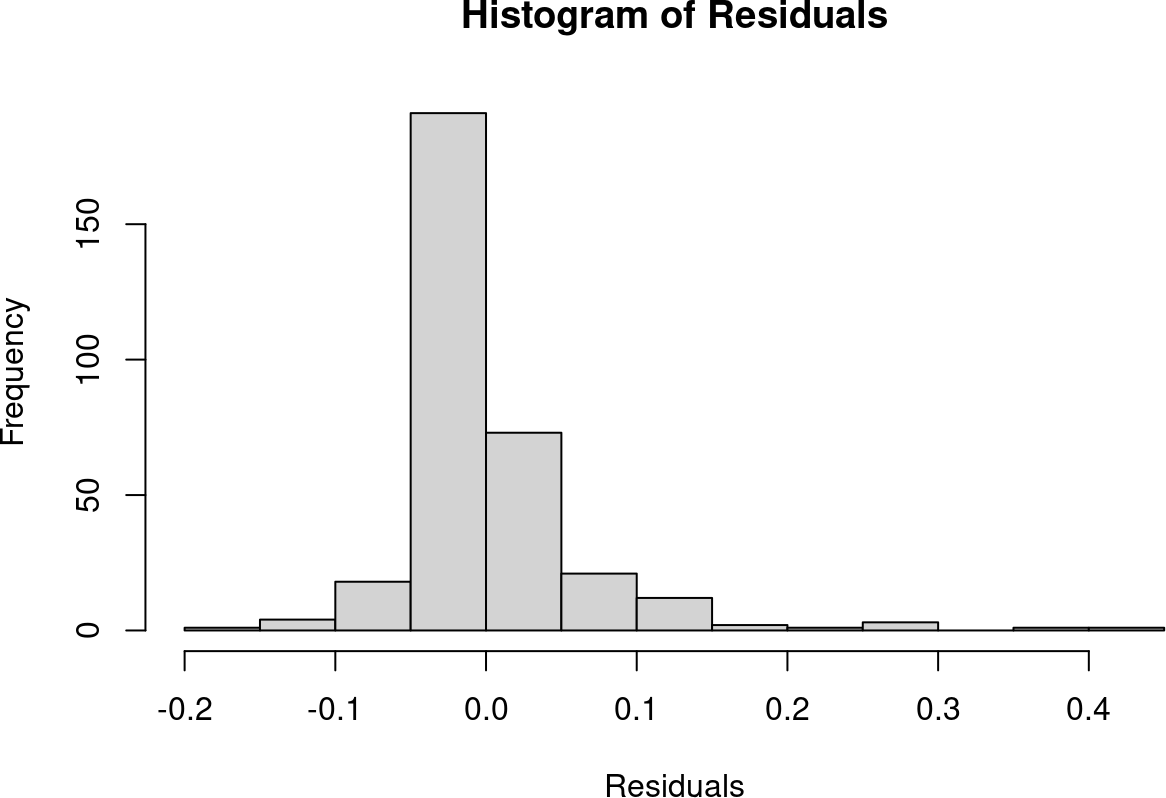#> #> Shapiro-Wilk normality test #> #> data: resids #> W = 0.75853, p-value < 2.2e-16 With a p-value less than 0.05 for the Shapiro-Wilk test there is strong evidence that the residuals are not normally distributed and therefore violating the Gaussian assumption. A transformation of the response is warranted; a log transformation returns an acceptable Shapiro-Wilk p-value. Significant_SNPs <- preselection(Y = log(Y), SNPs = SNPs,number_cores = 1, principal_components = FALSE,frequentist = TRUE,controlrate = "bonferroni", threshold = .05,kinship = kinship,info = RealDataInfo) sum(Significant_SNPs$Significant)#4 Significant SNPs
#>  4
Significant_SNPs[Significant_SNPs$Significant == 1,c(1,2)] #> Chromosomes Positions #> 801 4 18532166 #> 820 4 18537303 #> 833 4 18540401 #> 852 4 18545882 With the new log transformed response there are now 4 significant SNPs from chromosome 4. resids_diag(Y = log(Y), SNPs = SNPs, significant = Significant_SNPs$Significant,kinship = kinship)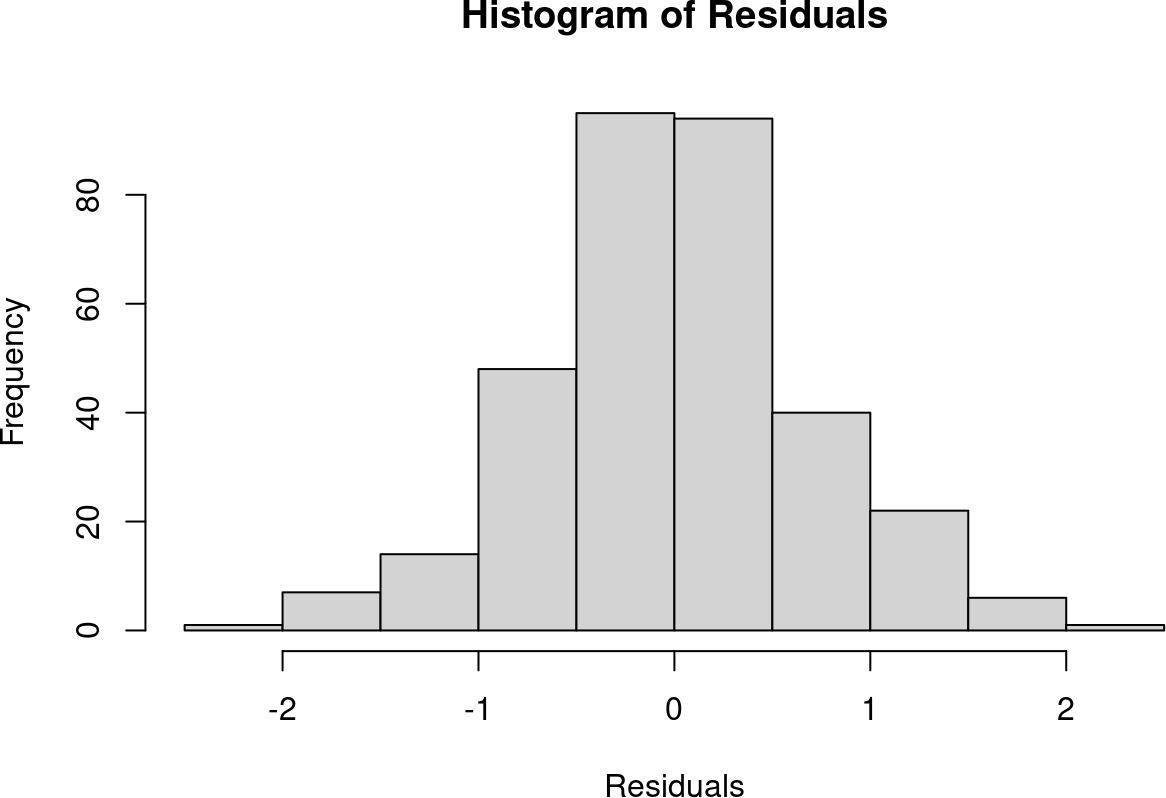#>
#>  Shapiro-Wilk normality test
#>
#> data:  resids
#> W = 0.99359, p-value = 0.1785

Now there is no evidence of violation of the Gaussian assumption. Therefore, we can trust the results.

## 5.3 Manhattan and QQ Plots using qqman package

If one would like to visualize the common Manhattan plots, the package qqman (Turner (2014)) is a great package for these plots.

library(qqman,quietly = TRUE)
manhattan(Significant_SNPs,chr = "Chromosomes",bp = "Positions",
p = "P_values",suggestiveline = FALSE,
genomewideline = -log10(.05/nrow(Significant_SNPs)))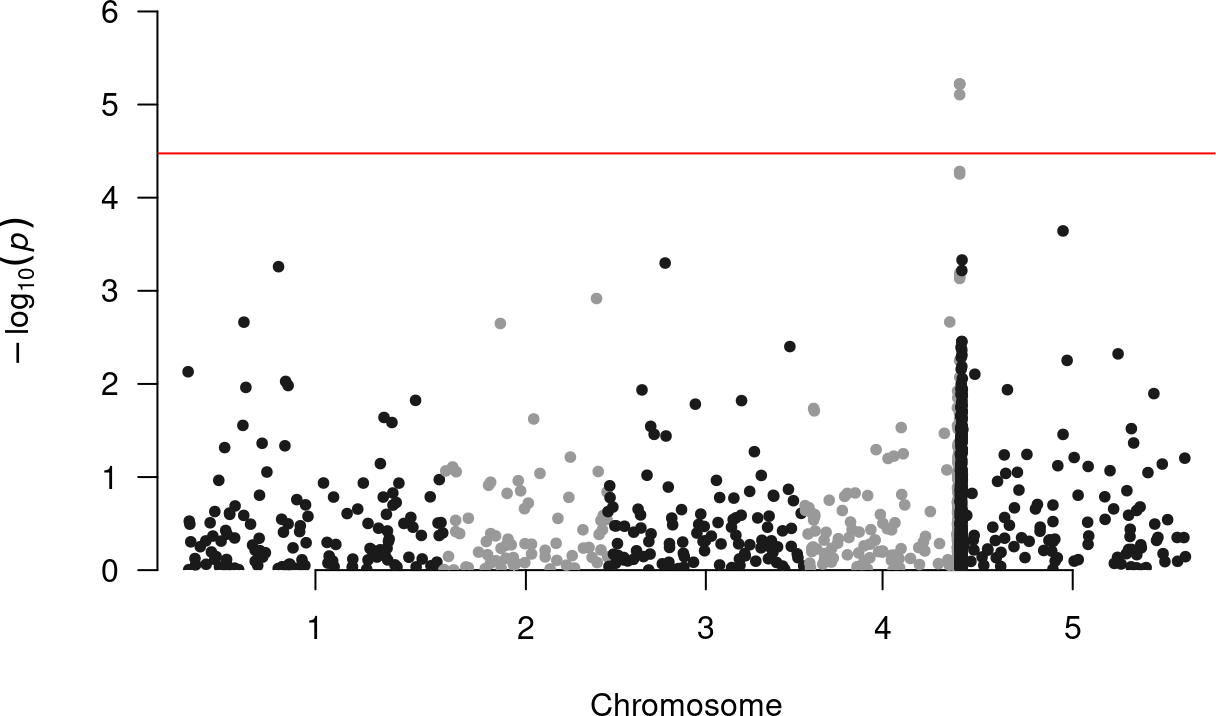The different colors in the manhattan plot highlight different chromosomes.

The qqman package can also make QQ plots for the p-values with the function qq. The function plots the expected value (under the null hypothesis of no SNP effect) of -log10(p-value) in the x-axis and the observed p-value in the y-axis. Departures from a straight line indicate that there are SNPs that are predictive of the phenotype. Here is how to use the qq function:

qq(Significant_SNPs$P_values)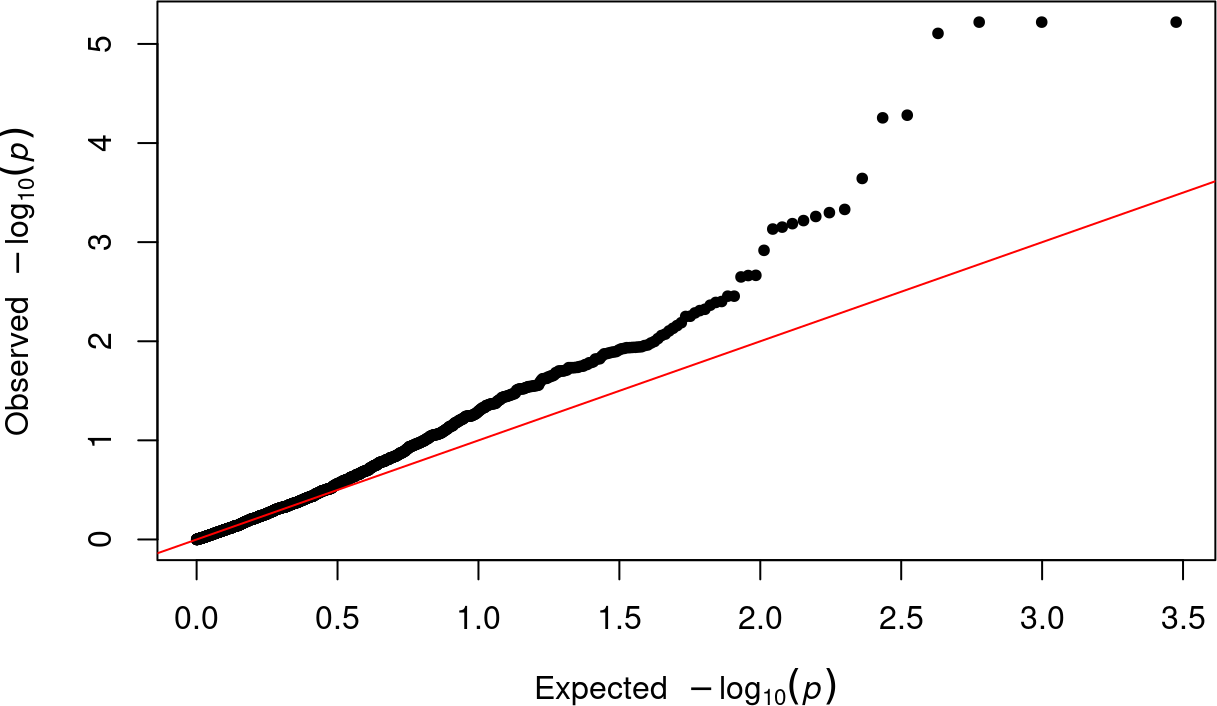Because the line deviates from a straight line, there is evidence that there are SNPs that are predictive of the ratio of average lateral root and main root length at low salt stress levels. ## 5.4 Bayesian Model Selection Next we perform Bayesian model selection to identify, amongst these 4 SNPs, which ones are important for predicting the phenotype. GA_results <- postGWAS(Y = log(Y),SNPs = SNPs,number_cores = 1, significant = Significant_SNPs$Significant,principal_components = FALSE,
kinship = kinship,info = RealDataInfo)
#>  "Dropped Columns: 3"
#>  "Dropped Columns: 3"
#>  "Dropped Columns: 3"
#>  "Dropped Columns: 3"
#>  "Dropped Columns: 3" "Dropped Columns: 4"
#>  "Dropped Columns: 4"
#>  "Dropped Columns: 4"
#>  "Dropped Columns: 3" "Dropped Columns: 5"
GA_results
#> $Models #>  "Model 1: y ~ SNP833 Posterior Prob. of 0.0915" #>  "Model 2: y ~ SNP801 Posterior Prob. of 0.1253" #>  "Model 3: y ~ SNP820 Posterior Prob. of 0.1253" #>  "Model 4: y ~ SNP801 + SNP820 Posterior Prob. of 0.1253" #>  "Model 5: y ~ SNP852 Posterior Prob. of 0.1253" #>  "Model 6: y ~ SNP801 + SNP852 Posterior Prob. of 0.1253" #>  "Model 7: y ~ SNP820 + SNP852 Posterior Prob. of 0.1253" #>  "Model 8: y ~ SNP801 + SNP820 + SNP852 Posterior Prob. of 0.1253" #> #>$Solution
#>      4-18532166 4-18537303 4-18540401 4-18545882
#> [1,]          0          0          1          0
#> [2,]          1          0          0          0
#> [3,]          0          1          0          0
#> [4,]          1          1          0          0
#> [5,]          0          0          0          1
#> [6,]          1          0          0          1
#> [7,]          0          1          0          1
#> [8,]          1          1          0          1

With 4 candidate SNPs, there are 2^4 - 1 = 15 possible models. The reason 15 models do not show up in the above output is because the output is limited to showing models only in top cumulative 95% of the posterior probability. Because the number of candidate SNPs is 4 (<= 12), the function postGWAS performs an exhaustive search and computes the posterior probabilities for all 15 possible models. In this example, models 2 through 8 have the exact same posterior probability. One way to interpret this result is that each of these three SNPs (801, 820, and 852) provide the same information. Another way of looking at this result is that having 1 SNP vs having all 3 of these SNPs in the model makes no difference. This may indicate high correlations amongst these 3 SNPs.

The Bayesian model search shows us that the three SNPs provide the exact same information to the phenotype. That is why the Bayesian model selection proposes 7 different models. It tells us that having 1 SNP vs having all 3 SNPs makes no difference, they provide the same information. In a lab setting these three SNPs should be looked at and since these SNPs are in the same region this region should be a high priority for further investigation.

Let us investigate this further with a correlation plot.

cor_plot(SNPs = SNPs[,Significant_SNPs$Significant == 1], significant = c(1,1,1,1), info = RealDataInfo[,Significant_SNPs$Significant == 1])